2019-05-19 09:39:08 nanhuaibeian 阅读数 437

## 一、对数变换

对数变换的表达式`g = c*log(1+f)`，主要应用压缩动态范围
其中 c 是一个常数，f 是浮点数，
图像类似：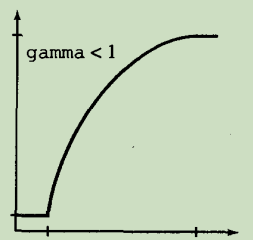gamma 曲线的形状可变，但是对数形状是固定的

当执行一个对数变换时，通常期望将导致的压缩值还原为显示的全范围

``````% 例如对于8比特而言
gs = im2uint8(mat2gray(g));
``````

使用函数 mat2gray 可将值限定在范围[0,1]内，使用函数im2uint8可将值限定在范围[0,255]内

示例：利用对数变换减小动态范围
题目：将一个取值范围在0至10^6的傅里叶频谱，显示在线性标度8位的显示系统上

``````>> g = im2uint8(mat2gray(log(1+double(f))));
>> imshow(g)
``````

这里之所以这么麻烦的转换：
是为了进行先归一化，然后转换成uint8，防止跨度过大的图像矩阵的边缘像素被抹去。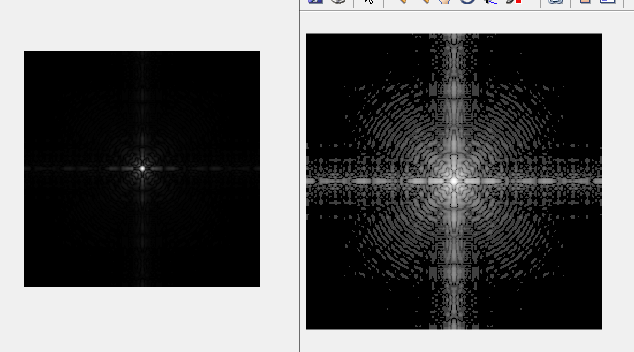## 二、对比拉伸变换函数

为什么要进行对比度拉伸变换 ？

对比度变换是一种通过改变图像像元的亮度值来改变图像像元的对比度，从而改善图像质量的图像处理方法。因为亮度值是辐射强度的反应，所以也称为辐射增强。
原理：将图像中过于集中的像元分布区域（亮度值分布范围）拉开扩展，扩大图像反差的对比度，增强图像表现的层次性。达到增强反差的目的，主要通过调整直方图来实现。

对比拉伸变换函数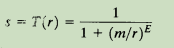`E = 20`时多得到的图像：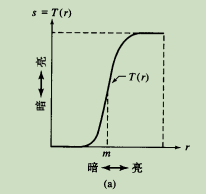其中r表示输入图像的亮度，s是输出图像的相应亮度值，E控制该函数的斜率
MATLAB中，该式由 `g = 1./(1+(m./(double(f)+eps)).^E)`，由于g的限制值为1，因此使用这种类型变换时输出值不能超过范围[0,1 ]
该函数可将窄范围的输入灰度级扩展为宽(拉伸)范围的输出灰度级，输出的是一幅具有高对比度的图像

图像处理 对比度拉伸变换 相关内容

2020-01-08 16:37:42 qq_38344751 阅读数 28

对比度拉伸变换  对图像进行对比度拉伸变换，压缩动态范围，将我们所关注的边界特征信息详细化，从而使得输出图像亮区域更亮，暗区域更暗，提高了图像的对比度。

opencv中的  LUT函数（look up table）为查表函数，

void LUT(InputArray src, InputArray lut, OutputArray dst);

InputArray src：输入图像

OutputArray dst：输出图像

InputArray lut：查找表

``````#include<opencv2/opencv.hpp>
#include<iostream>
#include<string>
#include<vector>
using namespace std;
using namespace cv;

void contrastStretch(Mat &img)
{
if (img.empty())
{
cerr << "image empty" << endl;
return;
}
// 计算图像的最大最小值
double pixMin, pixMax;
minMaxLoc(img, &pixMin, &pixMax);

//create lut table
Mat lookut(1, 256, CV_8U);
for (int i = 0; i < 256; i++)
{
if (i < pixMin)
lookut.at<uchar>(i) = 0;
else if (i > pixMax)
lookut.at<uchar>(i) = 255;
else
lookut.at<uchar>(i) = static_cast<uchar>(255.0*(i - pixMin) / (pixMax - pixMin) + 0.5);
}
LUT(img, lookut, img); //(look up table）即为查表函数
}

int main()
{
if (!srcImage.data)
return 0;
Mat resultImage;

vector<Mat> channel;
split(srcImage, channel);
contrastStretch(channel);
contrastStretch(channel);
contrastStretch(channel);
merge(channel, resultImage);//合并3个通道

imshow("srcImage", srcImage);
imshow("resultImage", resultImage);
waitKey(0);

return 0;
}``````

结果如下，图像变得更加对比清晰图像处理 对比度拉伸变换 相关内容

2018-12-18 10:23:11 qq_41881554 阅读数 1176

原理见《数字图像处理》第三版3.2.4

输出灰度和输入灰度的关系如下图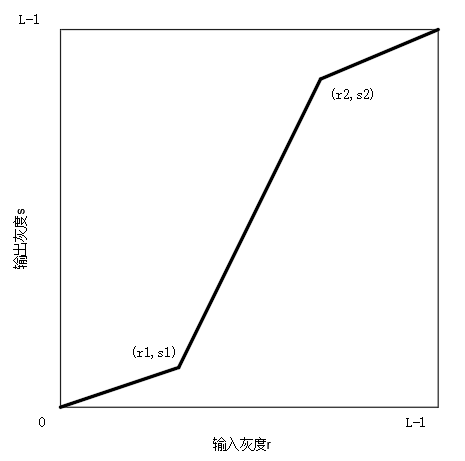现在令(r1,s1)=(rmin,0)；(r2,s2)=(rmax,L-1)和(r1,s1)=(m,0)；(r2,s2)=(m,L-1)，m为灰度均值

代码如下（VS2013+OpenCV3.0）

``````/*
//***************************************************************************************
//-----------------------------------------------作者：我三食堂不服-----------------------
//***************************************************************************************
*/

#include <opencv2/opencv.hpp>
#include <math.h>

using namespace std;
using namespace cv;

/*
//图像对比度拉伸。
//（r1,s1)灰度平面上的第一个点
//（r2,s2)灰度平面上的第二个点
*/
Mat Stretch(Mat input, int r1, int s1, int r2, int s2);

//获取图片灰度值最大值与最小值
int RmimRmax(Mat input, int &rmin, int& rmax);

//获取图像平均灰度值
int Average(Mat input);

int main(int argc, char** argv)
{
Mat im_source;			        	//原图
Mat im_pro;					//处理后的图片
Mat im_pro2;					//处理后的图片

//载入原图（灰度图方式）

//图片处理
int rmin{ 255 };
int rmax{ 0 };
RmimRmax(im_source, rmin, rmax);
im_pro = Stretch(im_source, rmin, 0, rmax, 255);
int m = Average(im_source);
im_pro2 = Stretch(im_source, m, 0, m, 255);

//创建窗口
namedWindow("【原图】");
namedWindow("【处理后】");
namedWindow("【处理后2】");

//显示图片
imshow("【原图】", im_source);
imshow("【处理后】", im_pro);
imshow("【处理后2】", im_pro2);

//保存图片
imwrite("3-10_c.tif", im_pro);
imwrite("3-10_d.tif", im_pro2);

//等待键盘操作
cvWaitKey(0);

return 0;
}

/*
//图像对比度拉伸。
//（r1,s1)灰度平面上的第一个点
//（r2,s2)灰度平面上的第二个点
*/
Mat Stretch(Mat input, int r1, int s1, int r2, int s2)
{
int h{ input.rows };				// 图片高
int w{ input.cols };				// 图片宽
Mat out{ h, w, CV_8UC1 };			// 输出图片
uchar* data_in{ input.data };		// 原图图片像素数据
uchar* data_out{ out.data };		// 输出图片像素数据
double data;						// 临时灰度值

double a, a1, a2, a3, b, b1, b2, b3;		// 直线斜率与截距
a1 = (double)s1 / (double)r1;
b1 = 0;
a2 = (double)(s2 - s1) / (double)(r2 - r1);
b2 = s1 - a2*r1;
a3 = (255.0 - s2) / (255.0 - r2);
b3 = s2 - a3*r2;

for (int y = 0; y < h; y++)
for (int x = 0; x < w; x++)
{
data = data_in[y*w + x];
if (data <= r1)
{
a = a1;
b = b1;
}
else if (data >= r2)
{
a = a3;
b = b3;
}
else
{
a = a2;
b = b2;
}
data = a*data + b;

data_out[y*w + x] = (uchar)(data);
}

return out;
}

//获取图片灰度值最大值与最小值
int RmimRmax(Mat input, int &rmin, int& rmax)
{
int h{ input.rows };				// 图片高
int w{ input.cols };				// 图片宽
uchar* data_in{ input.data };		// 原图图片像素数据

for (int y = 0; y < h; y++)
for (int x = 0; x < w; x++)
{
if (rmin>data_in[y*w + x])
rmin = data_in[y*w + x];

if (rmax < data_in[y*w + x])
rmax = data_in[y*w + x];

}

return 0;
}

//获取图像平均灰度值
int Average(Mat input)
{
long long re{ 0 };
int h{ input.rows };				// 图片高
int w{ input.cols };				// 图片宽
uchar* data_in{ input.data };		// 原图图片像素数据

for (int y = 0; y < h; y++)
for (int x = 0; x < w; x++)
{
re += data_in[y*w + x];
}
re /= (h*w);

return (int)re;
}``````

效果如下：

图像处理 对比度拉伸变换 相关内容

2011-11-23 15:31:18 honesty2008 阅读数 8874

实验题目:

根据下图参考数据和图像，编程实现数字图像的对比度拉伸。实验分析:

根据上图，可得：我们只要把图形中灰度值为28-75的像素点修改灰度值即可以完成本次实验。
可以先找出28，75的纵坐标，根据两点式求出该范围内的直线方程。
如设两点坐标为（x0,y1）,(x1,y1),则斜率为(y1-y0)/(x1-x0)。

实验程序: lab1.m

```function lab1(addr,x1,y1,x2,y2)
%功能：完成实验一，灰度拉伸处理
% x1,y1,x2,y2 为拉伸处理的线段端点坐标，当参数小于5个时
% 这些坐标均采用默认值，可以直接按 F5 运行。
if nargin<1
end
if nargin<5
x1=28;y1=28;
x2=75;y2=255;
end
if  x1==x2
display('x1、x2不能相同，退出程序');
return ;
end
[r,c,n]=size(I);
k=(y1-y2)/(x1-x2);
b=y1-k*x1;
if n>1
I2=rgb2gray(I);
else I2=I;
end
I2=double(I2);
for x=1:r
for y=1:c
tmp=I2(x,y);
if tmp>=x1 && tmp<=x2
I2(x,y)=k*tmp+b;
end
end
end
subplot(1,2,1);imshow(I);title('original image ');
subplot(1,2,2);imshow(I2,[]);title('image after modification');
```

图像处理 对比度拉伸变换 相关内容

2016-08-04 20:17:28 hhw999 阅读数 4253

对数及对比度拉伸变换

对数和对比度拉伸变换是：动态范围操作的基本工具

表达式：g=c*log(1+f)，其中c是一个常数，f是浮点数

对数变换：
应用：压缩动态范围（实现了图像灰度扩展和压缩功能，扩展低灰度值而压缩高灰度值，让图像的灰度分布更加符合人的视觉特性）（灰度值0（黑）~255（白））
可以利用这种变换来扩展被压缩的高值图像中的暗像素

mat2gray(f)：会将压缩值限定在【0,1】范围

im2uint8( mat2gray(f) )：会将值限定在【0,255】范围

对比度拉伸变换函数：把窄的输入灰度级扩展为宽的范围的输出灰度级，结果是一幅高对比度的图像
表达式：                  1
s=T(r)=   - - - - - - -     ，
1+(m/r)^E
其中r为输入图像的灰度，s是输出图像中的相应灰度值，E用于控制该函数的斜率

阈值化/阈值处理函数：用于图像分割的简单工具

图像处理 对比度拉伸变换 相关内容

阅读数 406

博文 来自： qq_43685640

阅读数 277

博文 来自： space_walk

阅读数 7022

博文 来自： Ibelievesunshine

阅读数 12972

博文 来自： qq_15096707

阅读数 1907

博文 来自： cyf15238622067
没有更多推荐了，返回首页